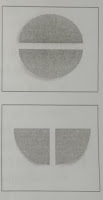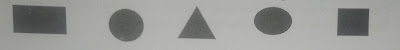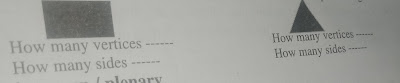# Lesson Plan of Geometry

Lesson Plan of Geometry (Two, Three – Dimensional Figures)

Subject Mathematics

Students` Learning Outcomes

• Identify the figures like square, rectangle, triangle, circle, semi-circle and quarter circle.
• Identify vertices and sides of a triangle, rectangle and square.

Information for Teachers

• Students already have a good idea of different basic shapes.
• Circle and oval shapes have no corners.
• Square and rectangle have four corners and four sides.
• Triangle has three corners and three sides.
• A vertex is the corner or intersection of any shape. The plural is vertices.
• The competency here is to develop mathematical vocabulary e.g. corners, vertices, sides, semicircle, circle, quarter circle,• While teaching the lesson, the teacher should also consult textbook at all steps wherein and whenever it is applicable.

Material / Resources

Writing board, chalk/marker, duster, glass, card sheet, scissors, 2 D shapes (triangle, rectangle, and square)

Introduction

• Ask the following questions from students to generate whole class discussion.
• What is the shape of the black board of your classroom?
• Identify the corners in this board.
• How many sides does this board have?
• Show them one note book, ask about its shape, corner and the number of sides it has.
• Repeat the same with different objects of different shapes.

Development

 Activity 1 Demonstrate to the students how they can get a circle, semicircle, and quarter circle by using a card sheet. Take a piece of paper and draw a circle with the help of a glass. Cut this circle with the help of scissors.Show and tell them that this is called circle. Ask one of the students to fold this circle into two halves. Now open the circle and cut it into two equal parts along the folding line. You may refer back to the concept of fractions at this point. You may also remind them that each circle is ½ or half of the original circle. Show and tell them that each part is called a semi-circle.Ask two of the students to take a semi-circle and fold it into two halves. Cut this semi-circle into two equal parts along the folding line. Show and tell them that each part is known as a quarter circle.You may remind them of fractions at this point. Remind them that in fraction terms a quarter is written as ¼ Sum up the demo by explaining that a circle equally divided in 2 parts is 2 semi-circles and each of those 2 semi-circles further divided in to two is 4 quarters of the circle.

 Activity 2 Divide the class in groups four. Provide each group with different shapes, as;Tell each group to highlight / color corners in each shape. Give them some time for discussion within groups. Call any two groups to present their work for whole class by pointing out corners in each shape. Encourage other students to ask different questions from presenters to clear the concept further. Sum up the activity by explaining that a corner of any shape is called vertex, explain about the sides of shapes as well. Be sure about the use of mathematical vocabulary.

 Activity 3 Show a big box of square shape to the students (Carton) Ask them to tell how many sides and vertices it has? Encourage them to put a mark or a line on the vertex they have already counted so they don`t count it a second time. Facilitate / explain if they find difficulty in understanding and identification. Repeat the same procedures by showing them different 2D and 3D objects e.g. egg/ oval stone, plastic block etc.

 Activity 4 Divide the class in groups of four. Provide them different objects (2D, 3D) Tell them to identify and explain about the sides and vertices of those objects to each other within group. Sum up the activity by asking following questions: How many sides and vertices do a square and rectangular have? How many sides and vertices do a triangular have? How many sides and vertices does an oval circle have?

 Activity 5 Provide them a work sheet with different shapes drawn on it, or draw these on the board for the students to copy. Ask the students to write how many sides and vertices does each shape have e.g.Sum up / Conclusion

• Ask the following questions from students, as;
• What was the lesson about?
• What is the appropriate word for corner? (Mathematical vocabulary)
• Can any one of you explain how many sides and vertices your teacher`s table has?
• How many sides and vertices does your classroom have?

Assessment

• Tell the students to draw different shapes e.g. square, rectangle, triangle, and circle, semi-circle and quarter circle in their notebooks / copies.
• Ask them to color their vertices and write how many sides each shape has?